A solenoidal coil with 25 turns of wire is wound tightly around another coil with 320 turns. The inner solenoid is 23.0cm long and has a dia

Question

A solenoidal coil with 25 turns of wire is wound tightly around another coil with 320 turns. The inner solenoid is 23.0cm long and has a diameter of 2.00cm . At a certain time, the current in the inner solenoid is 0.150A and is increasing at a rate of 1600A/sPart AFor this time, calculate the average magnetic flux through each turn of the inner solenoid.Part BFor this time, calculate the mutual inductance of the two solenoids;Part CFor this time, calculate the emf induced in the outer solenoid by the changing current in the inner solenoid.

in progress 0
6 months 2021-07-19T16:03:10+00:00 2 Answers 5 views 0

Explanation:

We have that the current in the inner solenoid is: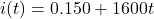A) the magnetic flux is given by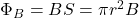B is obtained by computing for a solenoid: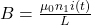hence, we have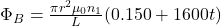B)

the mutual inductance is obtained by using: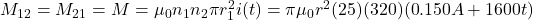C)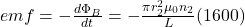hope this helps!!

2. Answer: Part A, magnetic flux = 9.02 × 10^-8 Wb

Part B, mutual inductance, M = 1.50 × 10^-5H

Part C, emf induced in the outer solenoid = -0.024V

Explanation: Please see the attachments below